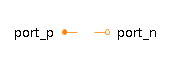Inverse Tangent 2 - MapleSim Help

Inverse Tangent 2

Output atan(u1/u2) of the inputs u1 and u2Description The Inverse Tangent 2 (or Atan 2) component computes, element-wise, the inverse tangent of $\frac{\mathrm{u1}}{\mathrm{u2}}$. The Signal Size parameter assigns the dimension of the input and output connectors.Equations ${y}_{i}=\mathrm{atan}\left({\mathrm{u1}}_{i},{\mathrm{u2}}_{i}\right)$Connections

 Name Description Modelica ID $\mathrm{u1}$ Real input vector u1 $\mathrm{u2}$ Real input vector u2 $y$ Real output vector yParameters

 Name Default Units Description Modelica ID Signal Size $1$ Dimension of input and output signals signalSizeModelica Standard Library The component described in this topic is from the Modelica Standard Library. To view the original documentation, which includes author and copyright information, click here.